# Aim of the experiment¶

1. To find the moment of inertia of belan

2. To find the relation ship between rolling time and the inclination angle

3. To find the coefficient of friction of the surface.

4. To determine if rolling time is dependant of surface friction.

# System description¶

Consider the inclined plane in figure 1.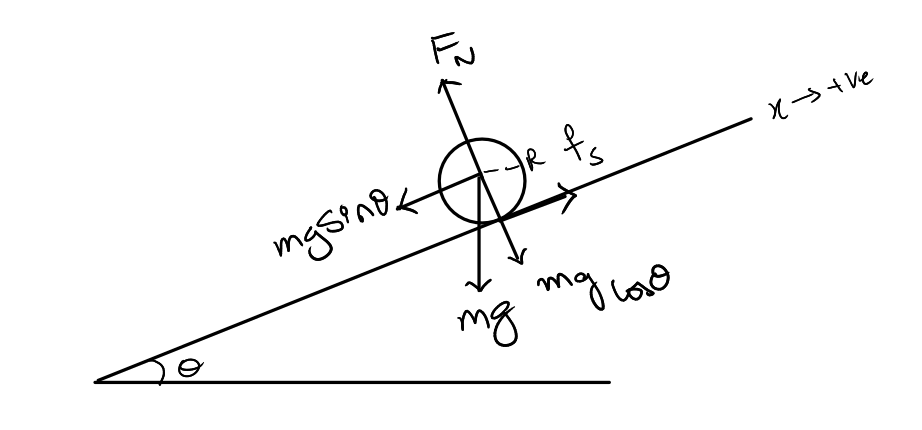let’s establish all the forces acting on this rolling body . The force mg is acting in the downward direction and it has two components one is parallel to the plank and there’s one component which is perpendicular to the plank and this in turn causes normal reaction and we call it $$F_{n}$$ .

We also have a force of friction which is acting opposite to the direction of roll. we are considering a situation where the body is not slipping down the ramp it is smoothly rolling down the lamp so force of friction which is static in nature is more than $$mg\sin\theta$$ and it is not necessary that $$f_{s}$$ here is $$f_{s}max$$, so the moment $$mg\sin\theta$$ exceeds $$f_{s}max$$ the body will start slipping (i.e for smooth rolling $$f_{s}>mg\sin\theta$$)

Lets write the equations of motion of this rolling body, first we’ll write the forces acting on the body considering its linear motion we can say

$$f_{s}-mg\sin\theta=ma_{x}$$

We now notice that only $$F_{s}$$can produce torque to the body as all other forces are going right through the axis of rotation of the cylinder and therefore its distance from the axis of rotation of the cylinder is zero .

$$\tau=I\alpha$$
$$f_{s}R=I\alpha$$
$$a_{x}=R\alpha$$
$$f_{s}=Ia_{x}/R^{2}$$
$$Ia_{x}/R^{2}-mg\sin\theta=ma_{x}$$
$$a_{x}=\frac{g\sin\theta}{1+I/mR^{2}}$$

The time taken for the cylinder to cover a distance S (along the inclination) can be calculated from

$$t=\sqrt{(\frac{2S}{a_{x}})}$$
$$t=\sqrt{\frac{2S(1+I/mR^{2})}{g\sin\theta}}\label{eq:time}$$

In the limiting case where frictional force can no longer support rolling of the cylinder, thus the cylinder begins to slip. Analysing this case, $$f_{s}=\mu N=\mu mg\cos\theta$$ thus

$$\mu mg\cos\theta_{max}=Ia_{x}/R^{2}$$
$$\mu mg\cos\theta_{max}=\frac{Ig\sin\theta_{max}}{R^{2}+I/m}$$
$$\mu=\frac{I\tan\theta_{max}}{mR^{2}+I}\label{eq:mu}$$

# Experimental Method¶

1. Measure the dimensions such as lengths and radii of the Belan and record them with percentage errors using the accuracy involved with measuring tools

2. Measure the bench parameters in a similar way.

3. Calculate lift parameters ( vertical lift required for each degree).

4. Lift one end the bench using a Car Jack along with some wooden planks (ensuring the balance of the bench isn’t disturbed).

5. Plot t versus sin$$\theta$$ other quantities like t2, 1/t, 1/t2 etc. and try to get a linear plot.

6. find the moment of inertia of belan.

7. To determine if rolling time is dependant of surface friction.

8. Try find coefficient of friction.

9. Try to replace the surface and determine if rolling time is dependant of surface.

# Observations & Measurements¶

## Measurement of linear distances¶

### Measurement of radii of belan¶

In order to measure the radii of the belan, a thread was used cover the circumference and a marking is done with a pen on both of the strings at the same level as shown in Fig 2 and then the thread is placed on a ruler and the distance between the two markings on the string is measured, as shown in Fig 3 . The least count of scale is 0.05 cm for the smaller radius and 0.1cm for bigger radius.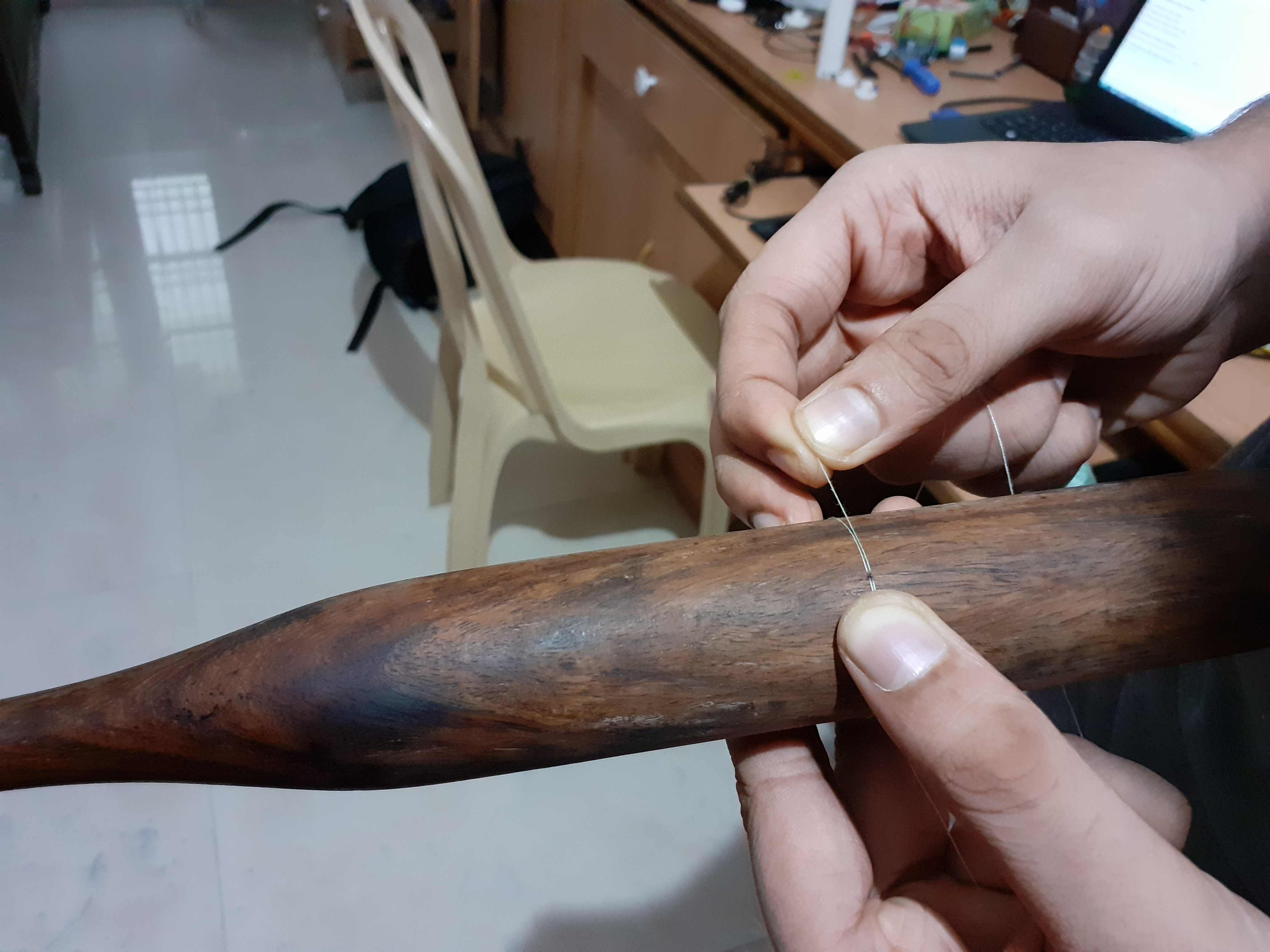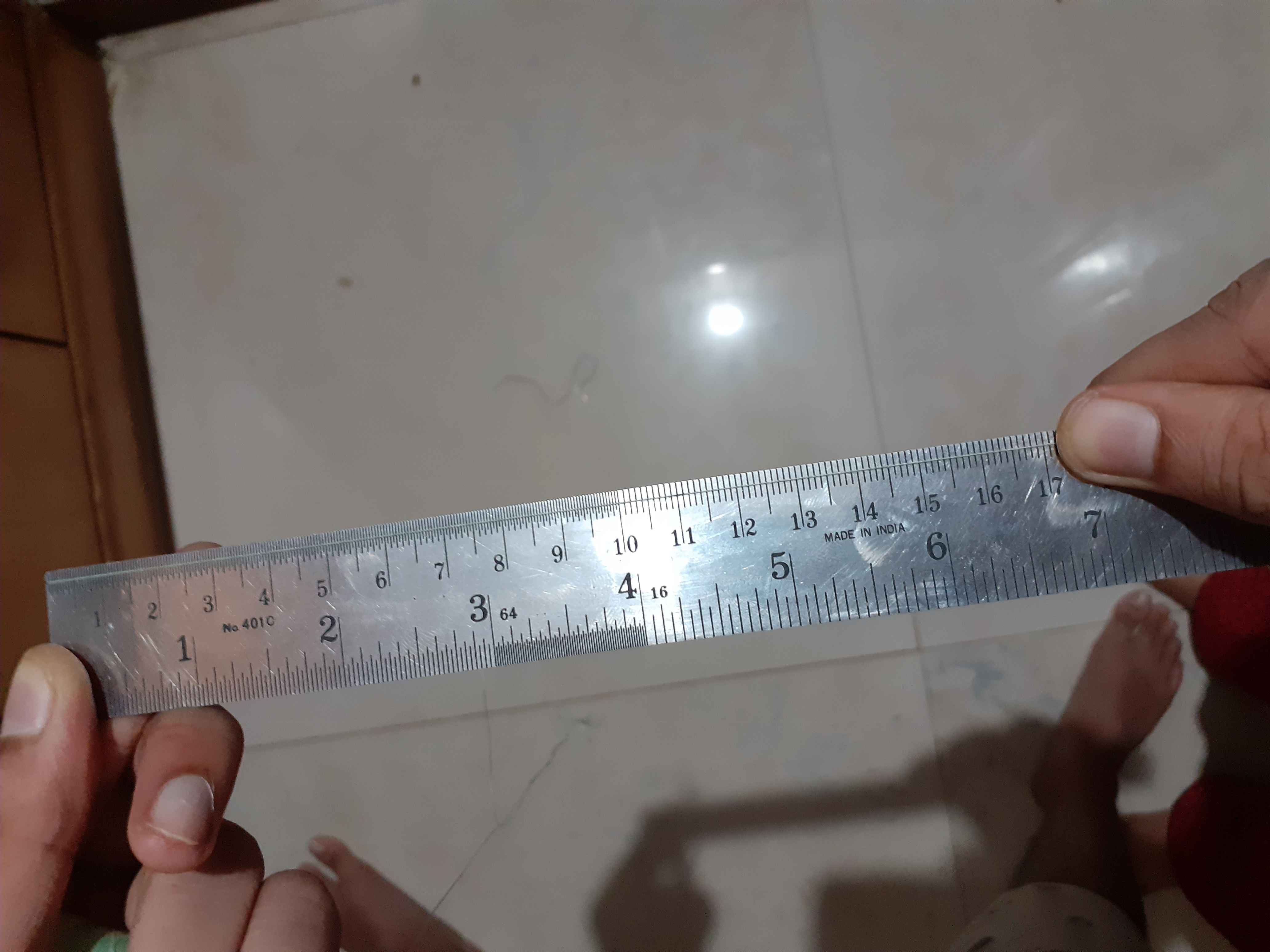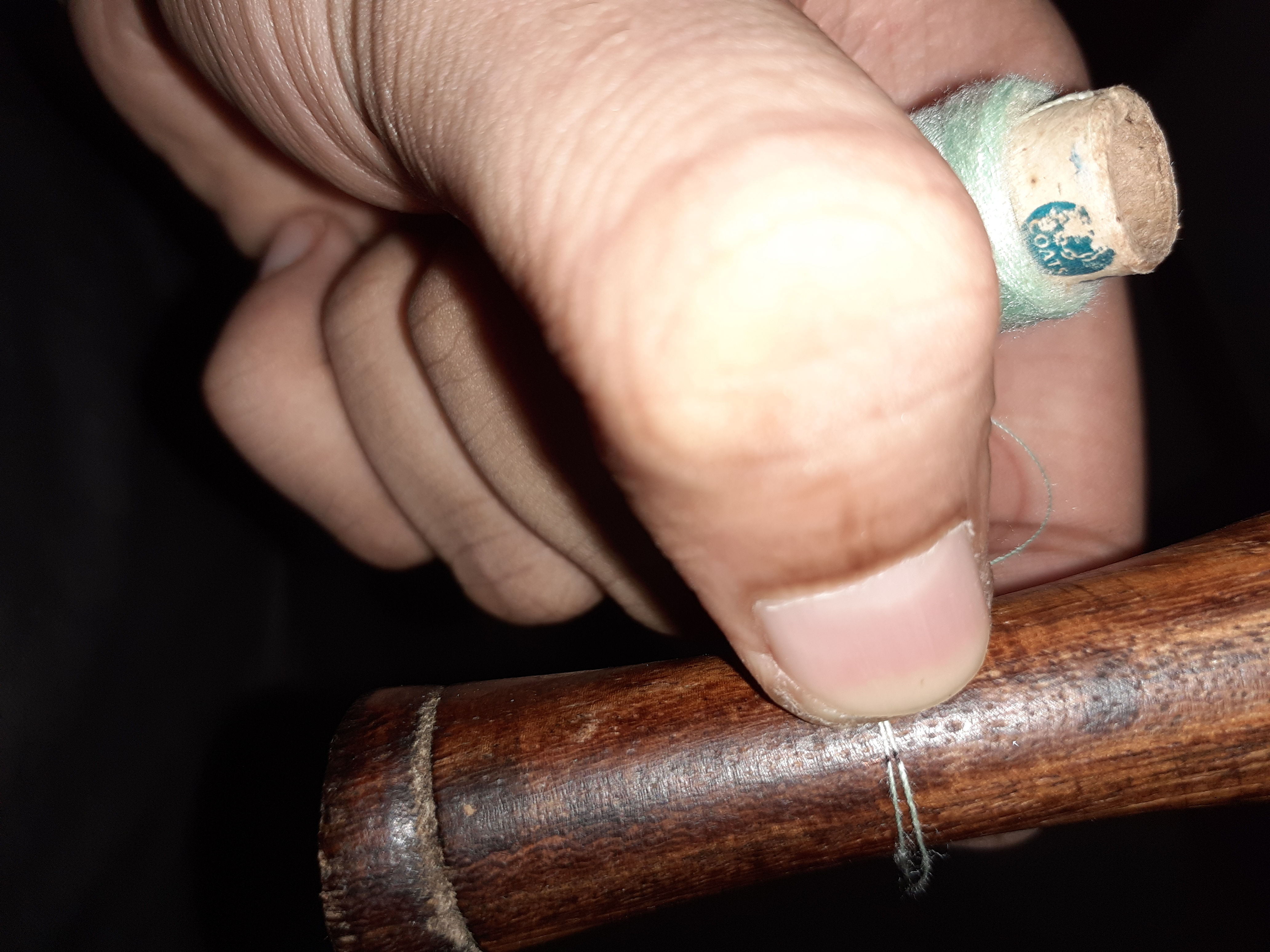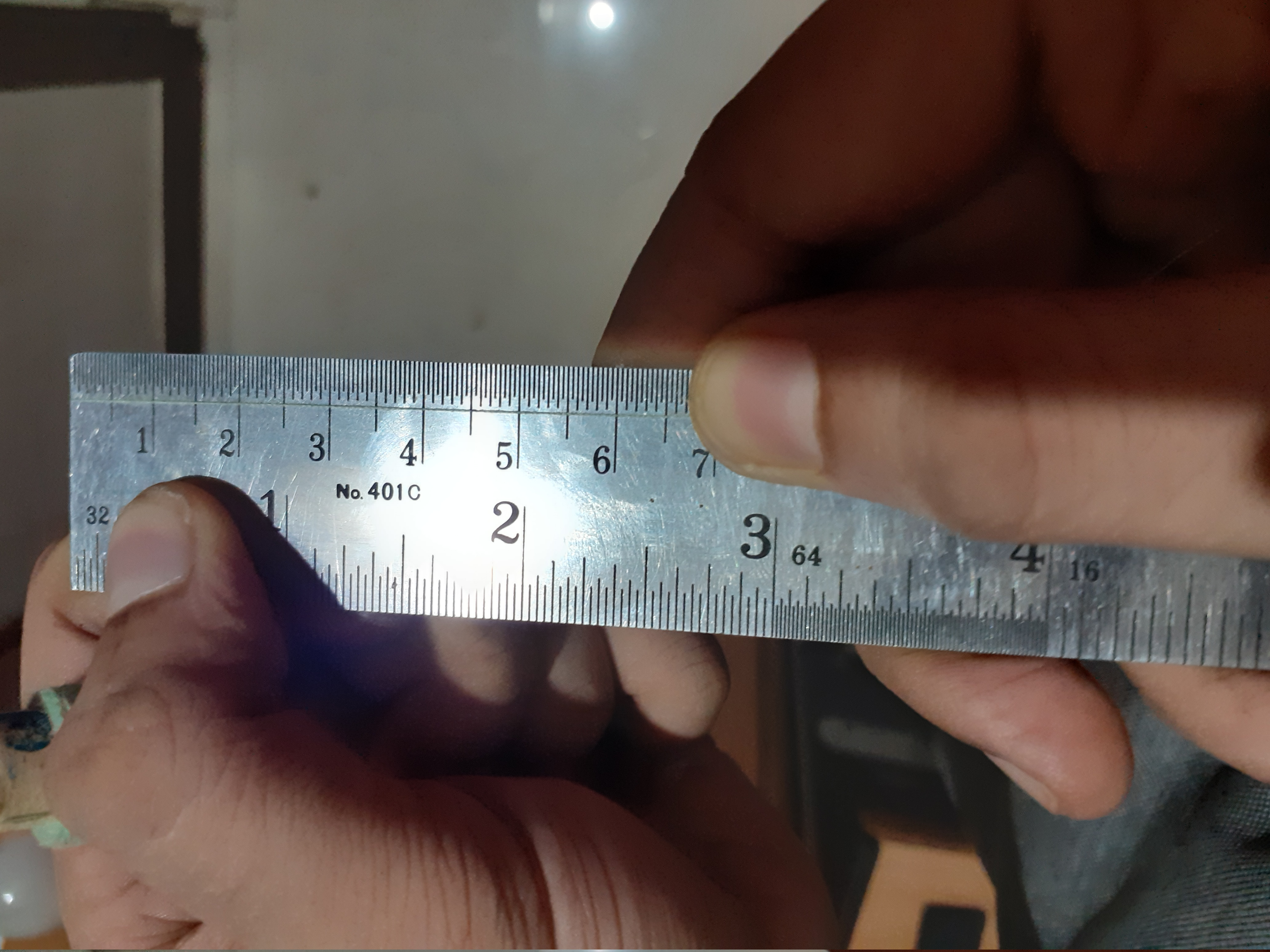Circumference of the smaller radius (in cm)   Circumference of the bigger radius (in cm)


Trial 1                         4.5                                           11
Trial 2                         4.6                                          11.2
Trial 3                         4.6                                          11.2

Mean value 4.56 11.13

Measured circumference data

Thus the nominal values of radii are 0.73 cm and 1.77 cm (round to two decimal places) respectively.

we know that $$c=2\pi r$$so, $$\Delta r=r\Delta c/c$$ so the error in measurement of radius is 0.008 cm and 0.016 cm respectively.

Therefore $$R_{1}=0.73\pm0.01$$cm and $$R_{2}=1.77\pm0.02$$cm (two significant figures).

### Measurement of length of belan¶

In order to measure the length of the belan, I placed the belan against a wall and the height was recorded on top of a painters tape (Fig 6 ), next we use a tape measure to measure the distance from ground to this marking (Fig 7 ).

The length of belan as measured using this procedure is 35.8 $$\pm$$0.1 cm (least count of tape measure is 0.1 cm)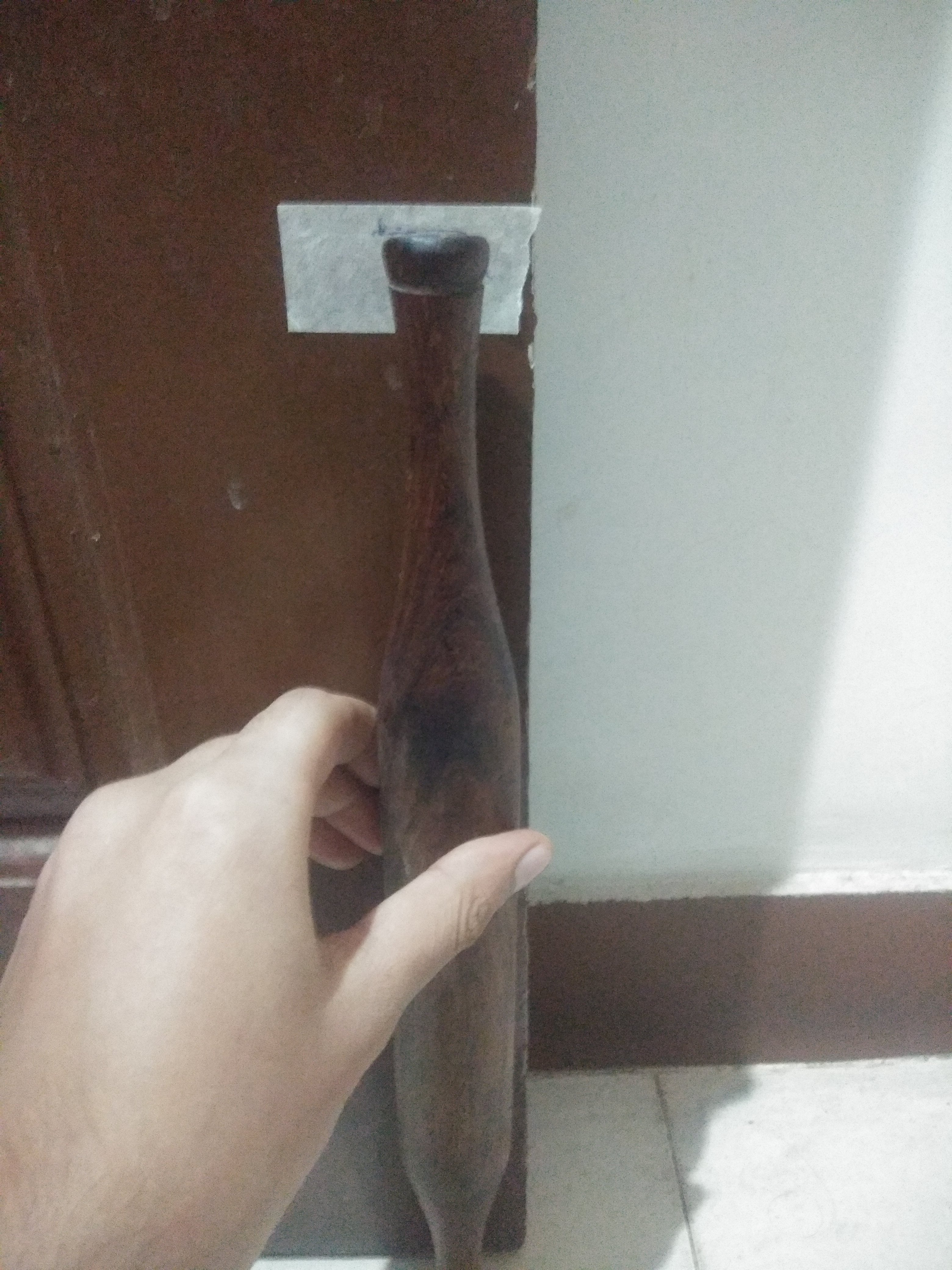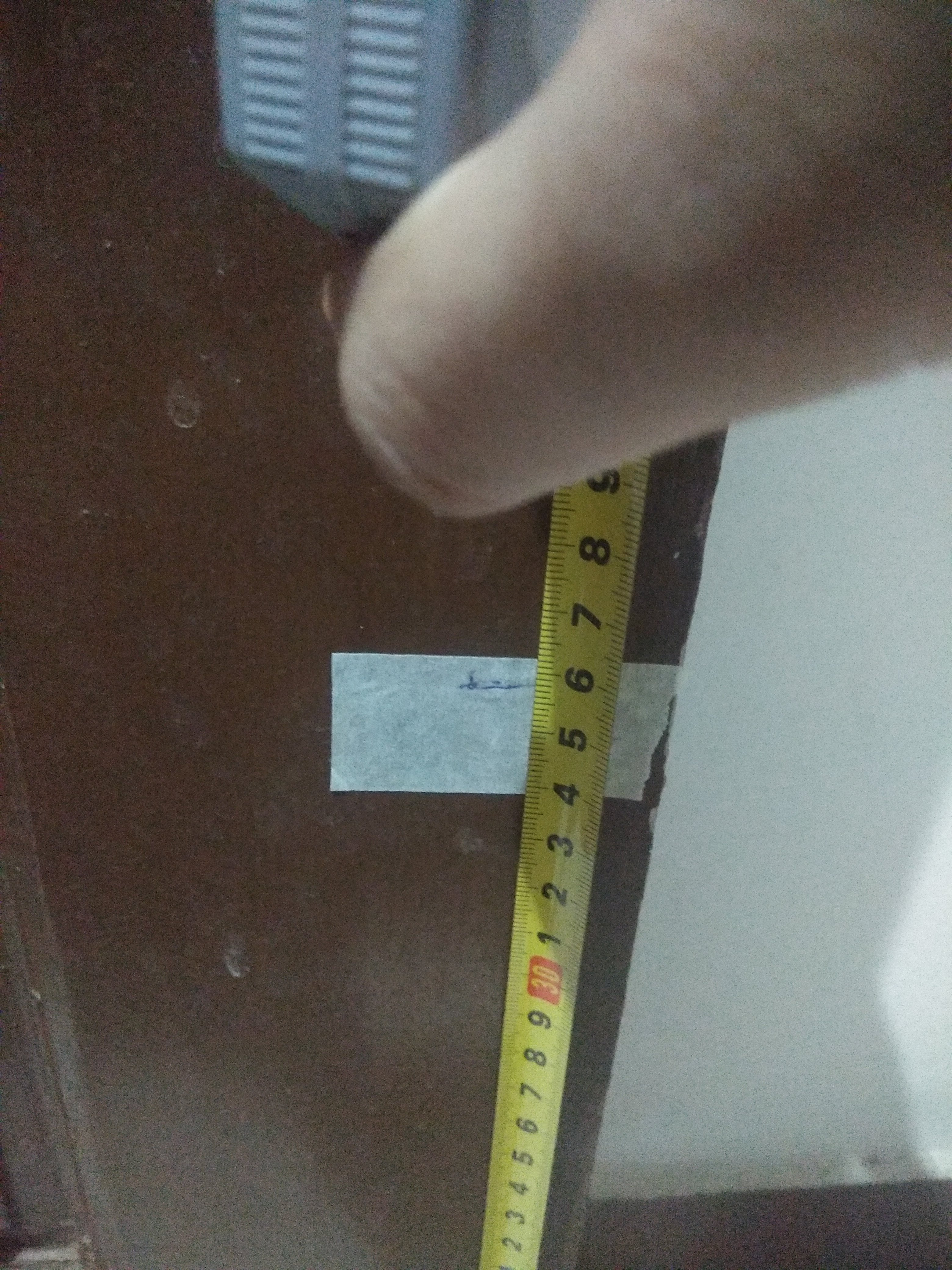### Measurement of distance travelled by belan¶

The bench used for the experiment has total length as 172cm (fig 8 ). However the belan can travel only part of the distance. On either side, the shape of the belan prevents it from starting at the very end (and reaching the lowest point) thus a tape has been put to keep track of the start and the end point of the belan. As seen from the Figure 10 & 9 , the total length the belan can traverse is (166 - 4.3) = 161.7 cm. The least count of the scale used here is 1mm. Therefore the total length the belan can traverse is 161.7$$\pm$$ 0.1 cm.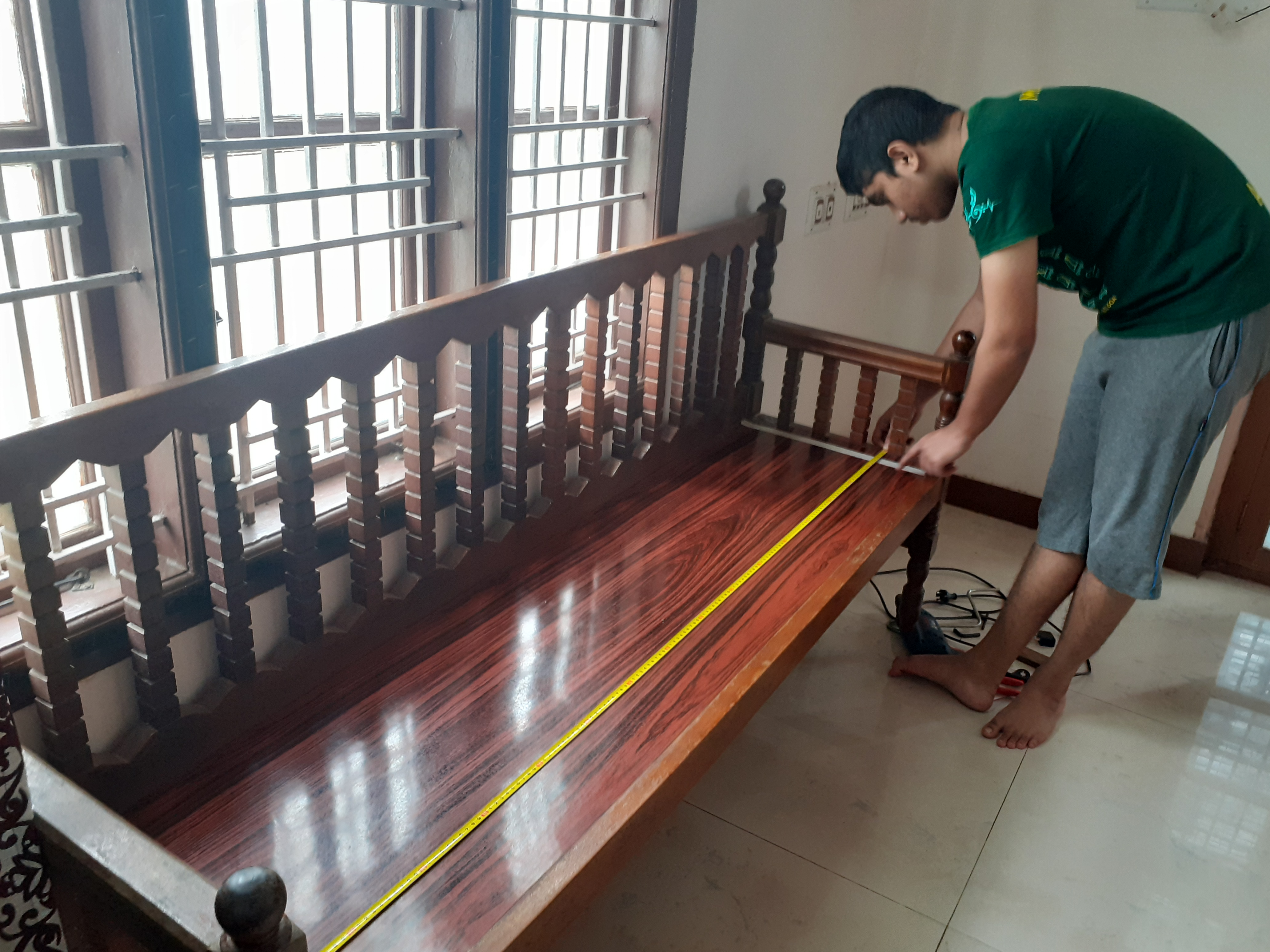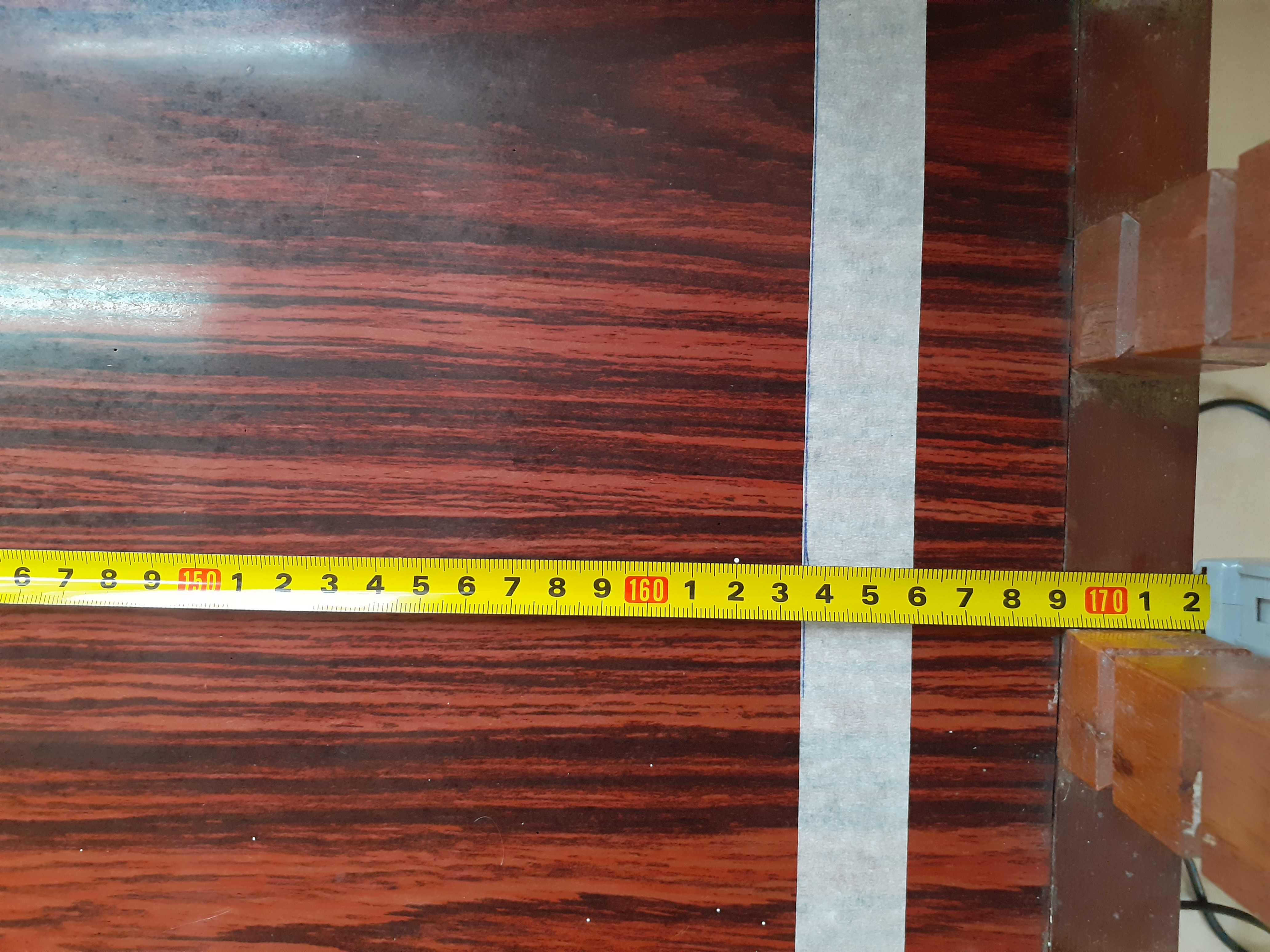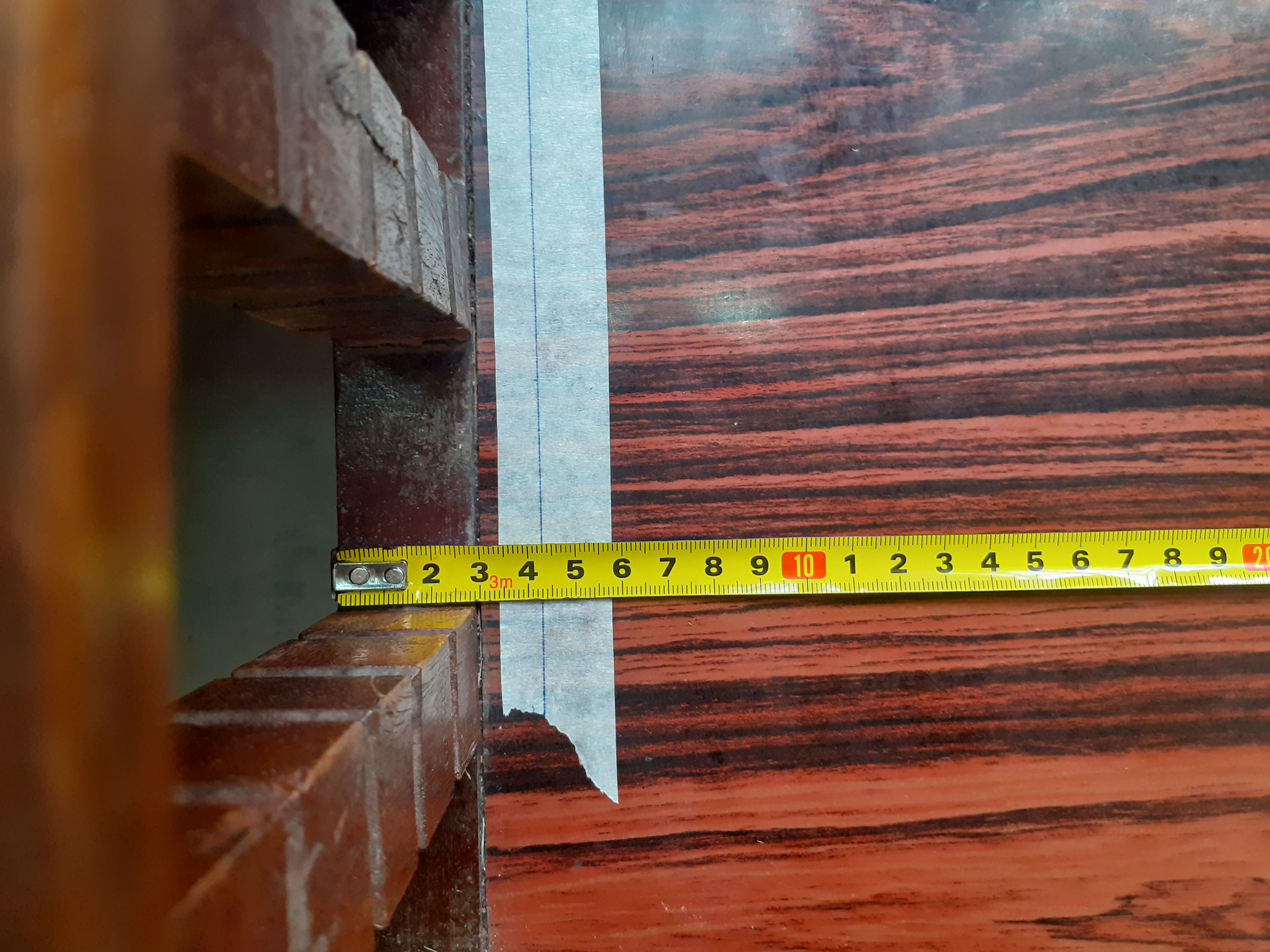### Measurement of vertical lift at one end¶

The selected bench for measurement has maximum flat length as 172 cm. We want to perform experiment at angles from 2 degree to 16 degree at an interval of 2. We can calculate the height required to be lifted at one end to reach the given set of angles as $$L=172\sin\theta$$. This gives us the following table 1 .

Angle(degree) lift required (cm) lift rounded to LC of scale (cm)

2 6.003 6 4 11.998 12 6 17.979 18 8 23.938 24 10 29.867 30 12 35.761 35.8 14 41.611 41.6 16 47.410 47.4

.

The Car Jack has been placed at the middle of one end of the bench (Fig12 ). For larger lengths (20cm onward) a set of wooden planks are added to increase the height (Fig 13). The bench can be lifted higher by rotating the shaft of the car jack until the required height is met (Fig ). Since the height can be measured to 0.1cm accuracy (LC of the scale used) this also translates into the accuracy for angle of the inclined plane. While measuring height care must be taken that the scale is perpendicular to ground and the height of the center of the bench leg is measured. One can use a flat card as a pointer and to avoid parallax. (Fig 11)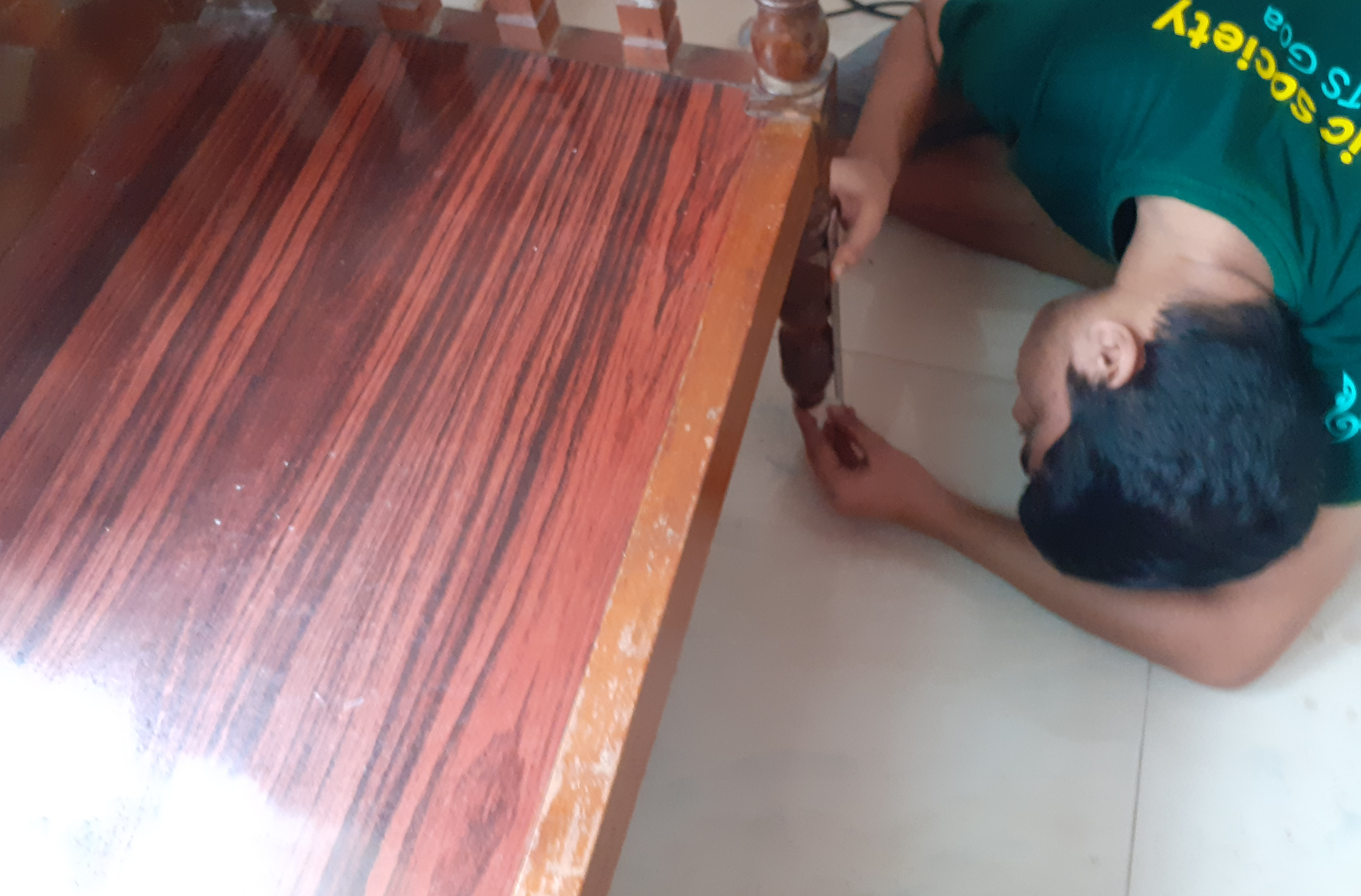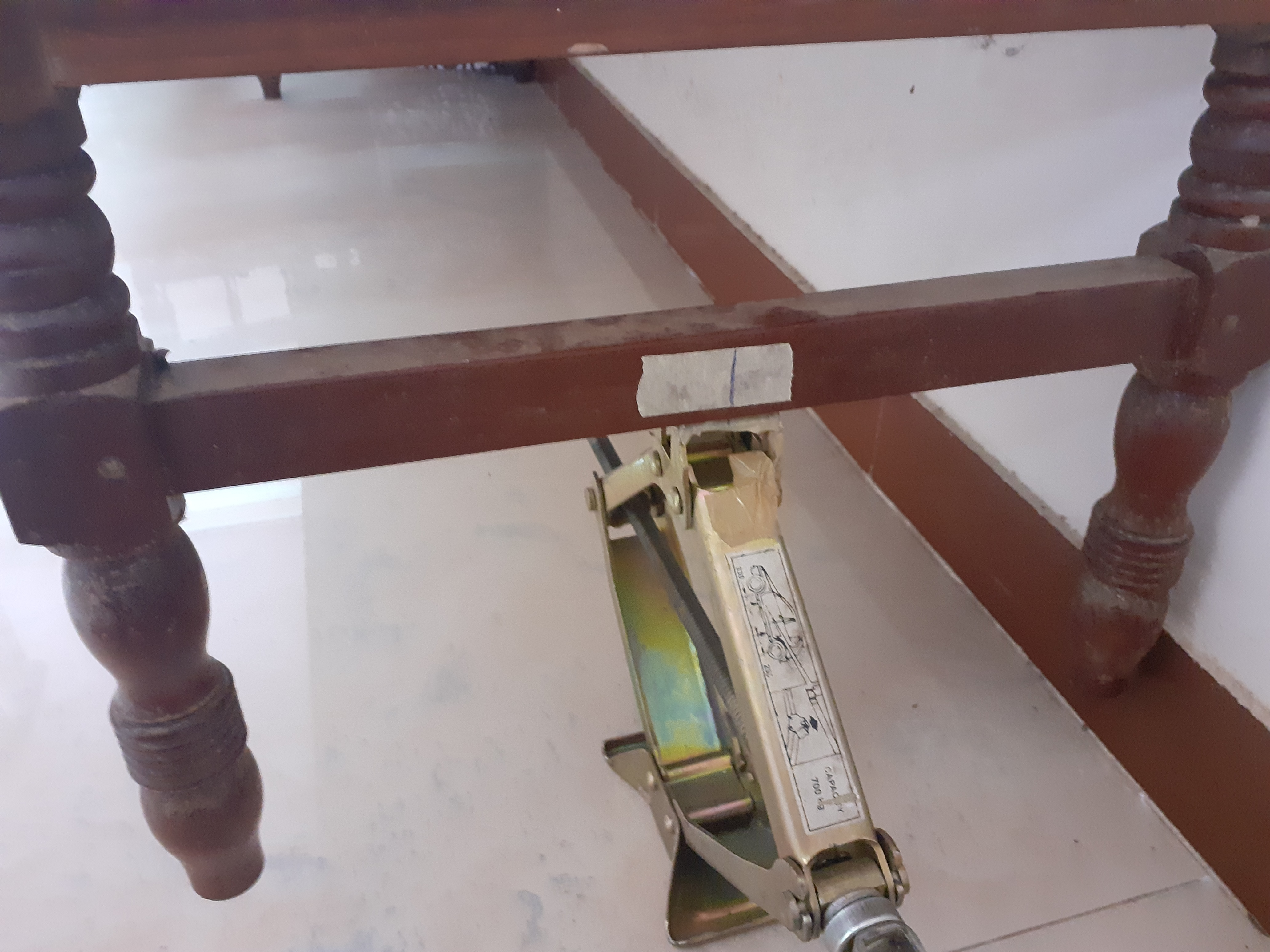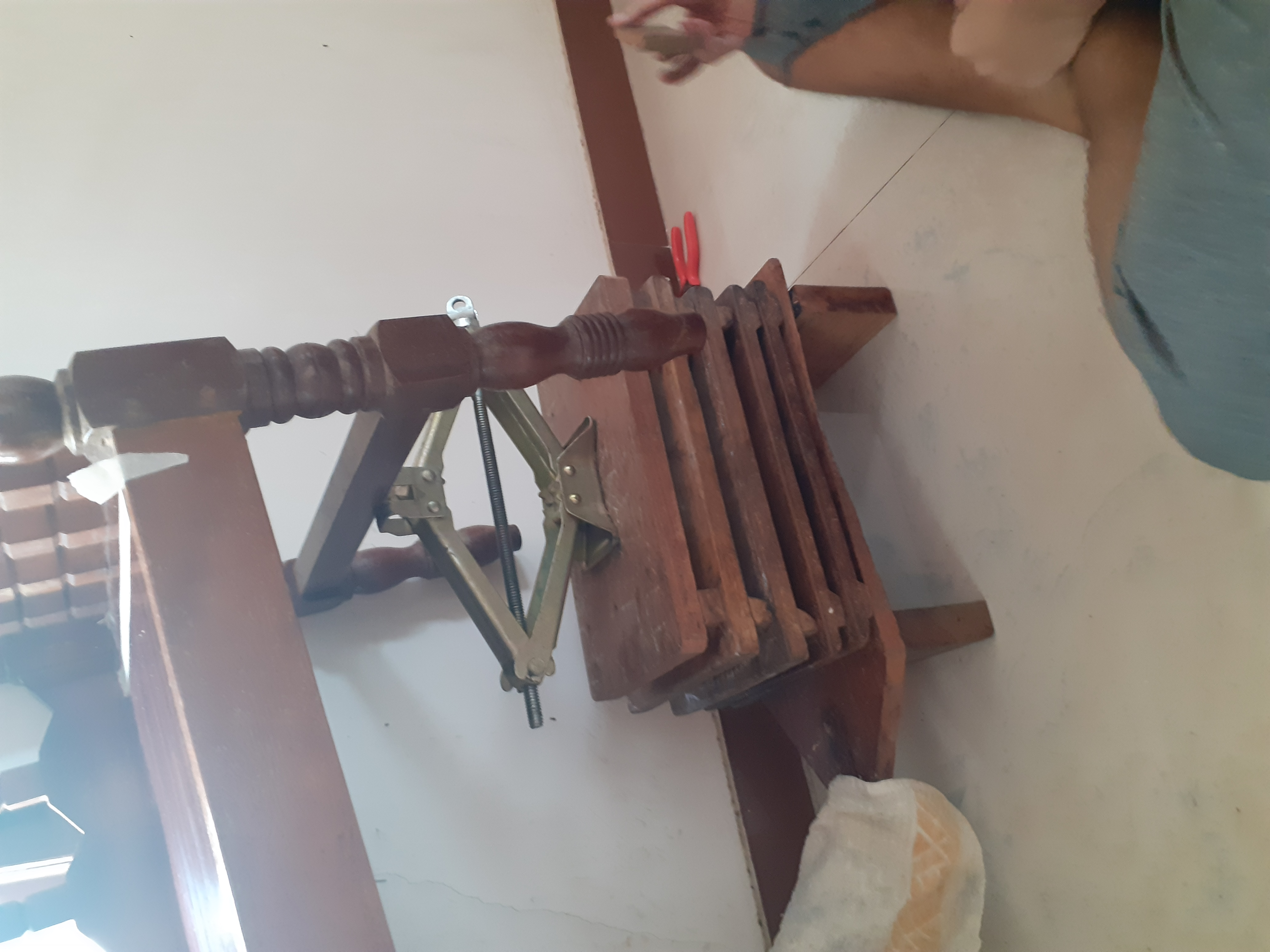## Measurement of time taken to roll down¶

In order to measure the time taken for the belan to roll down, video of the experiment was taken in a mobile phone. The video recorded was then processed frame by frame to find the number of frames between the starting and belan reaching the end. This analysis was done on a mobile app named Video Stopwatch (As seen in fig 14 ) .The video recorded had a 30 FPS (frames per second) thus each frame lasts for 33.33 ms and this is our least count. This exercises was done 3 times and the average was calculated.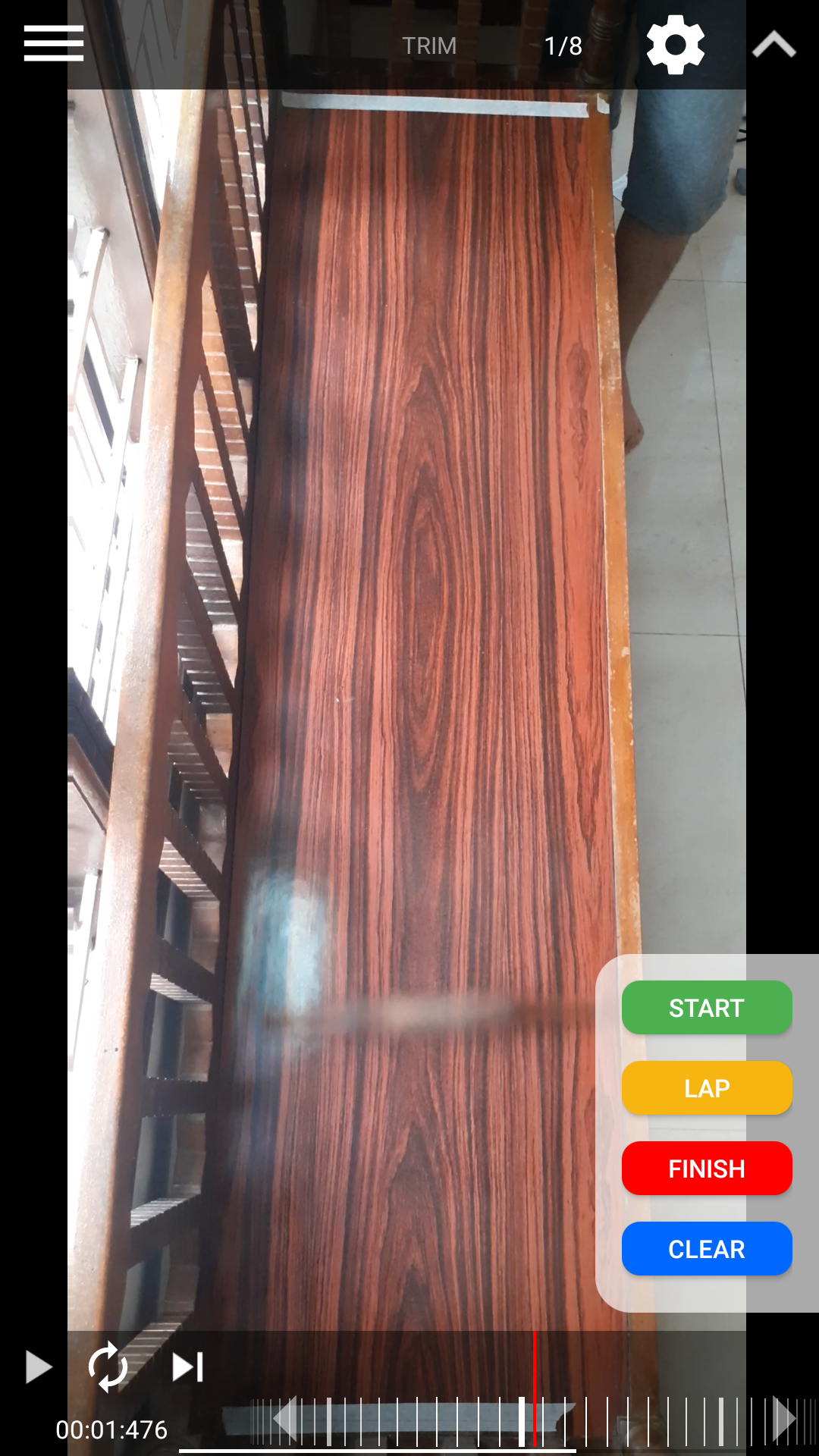Angle(degree)   time (trial1) (in s)   time (trial2) (in s)   time (trial3) (in s)   Mean time (in s)   lift of bench (cm)
--------------- ---------------------- ---------------------- ---------------------- ------------------ --------------------
2               3.5                    3.433                  3.433                  3.455              6
4               2.567                  2.567                  2.567                  2.567              12
6               2.067                  2.033                  2.033                  2.044              18
8               1.733                  1.8                    1.767                  1.767              24
10              1.633                  1.633                  1.633                  1.633              30
12              1.467                  1.433                  1.433                  1.444              35.8
14              1.3                    1.333                  1.333                  1.322              41.6
16              1.267                  1.2                    1.267                  1.245              47.4


After these sets of measurement, a kitchen anti slip mat was added on top of the bench surface. The time taken to roll down is measured again for 6 degree and 8 degree. The time measured thus is 2.033 s and 1.733 s respectively. This is similar to the one without surface modification thus rolling time is independent of surface (which is also clear from the formula for t Eq: [eq:time]).

## Measurement of weight of belan¶

The weight of the belan was calculated using a kitchen weighing scale.The least count of the used measuring scale was 1g. It was found that the weight of the belan used in this experiment is 172 $$\pm$$1 g.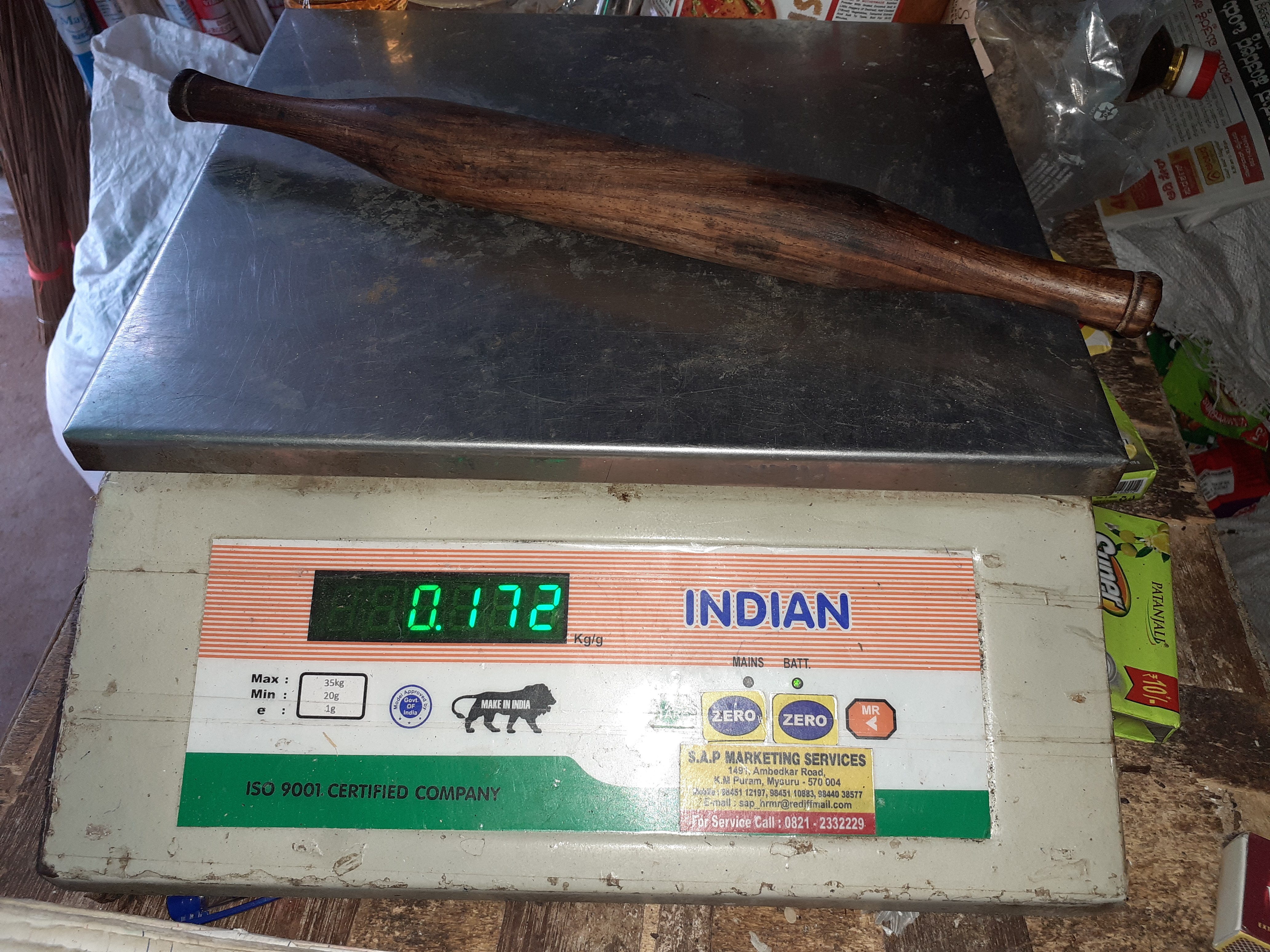## Plots¶

Attached outside the report in the form of 2 images.

## Calculation of moment of inertia of the belan¶

From eq [eq:time]

$$t=\sqrt{\frac{2S(1+I/mR^{2})}{g\sin\theta}}$$

so

$$I=mR^{2}(\frac{t^{2}g\sin\theta}{2S}-1)$$

let us take R as the weighted mean of $$R_{1}$$ and$$R_{2}$$. The weights can be chosen (assumed) as the fraction of total length that $$R_{i}$$ appears in the cylinder. The fraction of length with $$R_{1}$$ is 17/35.8 and that for $$R_{2}$$ is 18.8/35.8.

Thus R using these weights become $$\frac{aR_{1}+bR_{2}}{a+b}=1.28\pm(0.01+0.02)=1.28\pm0.03$$cm.

Using theses values and the vales from 2 degree angle data, and g=9.81m$$/s^{2}$$ we calculate I as 0.0000074312 Kg$$m^{2}$$ = 74.312 g$$cm^{2}$$

$$\frac{\Delta I}{I}=\frac{\Delta m}{m}+2\frac{\Delta R}{R}+2\frac{\Delta t}{t}+cos\theta\Delta\theta+\frac{\Delta S}{S}$$

calculating these with the previously mentioned error fractions,

$$\frac{\Delta I}{I}=0.073410$$
$$\Delta I=0.00000054552Kgm^{2}=5.45gcm^{2}$$

Therefore I = 74.312 $$\pm5.45gcm^{2}$$.

## Calculation of coefficient of friction¶

In the limiting case where frictional force can no longer support rolling of the cylinder, thus the cylinder begins to slip. In my case the $$\theta max$$was found to be 55 degree. Using eq[eq:mu]

$$\mu=\frac{I\tan\theta_{max}}{mR^{2}+I}$$

we get $$\mu=0.298\sim0.3$$

$$\frac{\Delta\mu}{\mu}=\frac{\Delta I}{I}+2\frac{\Delta R}{R}+\frac{\Delta m}{m}+cot\theta\Delta\theta$$
$$\frac{\Delta\mu}{\mu}=0.15473$$

Therefore $$\Delta\mu=0.046,$$thus $$\mu=0.298\pm0.046$$

# Results & Conclusion¶

1. The moment of inertia of belan is found to be 74.312 $$\pm5.45gcm^{2}$$

2. The relation ship between rolling time and the inclination angle is $$t=\sqrt{\frac{2S(1+I/mR^{2})}{g\sin\theta}}$$

3. The coefficient of friction of the surface is found to be $$\mu=0.298\pm0.046$$.

4. The rolling time is independent of surface friction (as long as $$f_{s}>mg\sin\theta$$).

Note : The above article is part of the report generated for regional NAEST Prelims.

So what do you think? Did I miss something? Is any part unclear? Leave your comments below.